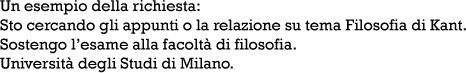# Esercizi in pascal

 Materie: Appunti Categoria: Informatica Download: 239 Data: 25.05.2000 Numero di pagine: 1 Formato di file: .txt (File di testo)
esercizi-pascal_3.zip (Dimensione: 0.94 Kb)
trucheck.it_esercizi-in-pascal.txt     1.7 Kb

Testo

{ Turbo Sort }
{ Copyright (c) 1985, 1989 by Borland International, Inc. }

program qsort;
{\$R-,S-}
uses Crt;

{ This program demonstrates the quicksort algorithm, which }
{ provides an extremely efficient method of sorting arrays in }
{ memory. The program generates a list of 1000 random numbers }
{ between 0 and 29999, and then sorts them using the QUICKSORT }
{ procedure. Finally, the sorted list is output on the screen. }
{ Note that stack and range checks are turned off (through the }
{ compiler directive above) to optimize execution speed. }

const
max = 10;

type
list = array[1..max] of integer;

var
data: list;
i: integer;

{ QUICKSORT sorts elements in the array A with indices between }
{ LO and HI (both inclusive). Note that the QUICKSORT proce- }
{ dure provides only an "interface" to the program. The actual }
{ processing takes place in the SORT procedure, which executes }
{ itself recursively. }

procedure quicksort(var a: list; Lo,Hi: integer);

procedure sort(l,r: integer);
var
i,j,x,y: integer;
begin
i:=l; j:=r; x:=a[(l+r) DIV 2];
repeat
while a[i]Il tuo nome: Scrivi le due cifre: